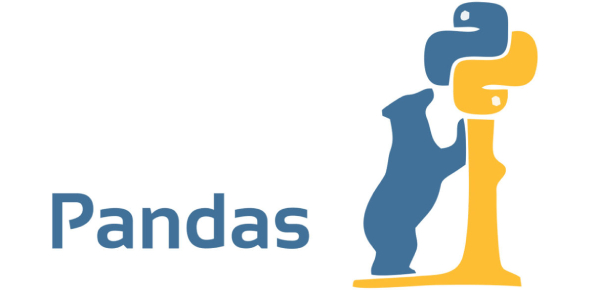# Quiz: How Much Do You Know About Python Panda?

10 Questions | Attempts: 830SettingsHow familiar are you with Python Pandas? If pretty much, you may already know that it a great choice for data science and analysis. To learn in detail, take the online quiz to see how well do you know Python Panda and learn more as you play.

• 1.
Which of the following thing can be data in Pandas?
• A.

A python dict

• B.

An ndarray

• C.

A scalar value

• D.

All of the mentioned

• 2.
Point out the correct statement.
• A.

If data is a list, if index is passed the values in data corresponding to the labels in the index will be pulled out

• B.

NaN is the standard missing data marker used in pandas

• C.

Series acts very similarly to a array

• D.

None of the mentioned

• 3.
Which of the following input can be accepted by DataFrame?
• A.

Structured ndarray

• B.

Series

• C.

DataFrame

• D.

All of the mentioned

• 4.
Point out the wrong statement.
• A.

A DataFrame is like a fixed-size dict in that you can get and set values by index label

• B.

Series can be be passed into most NumPy methods expecting an ndarray

• C.

A key difference between Series and ndarray is that operations between Series automatically align the data based on label

• D.

None of the mentioned

• 5.
All pandas data structures are ___ mutable but not always _______mutable.
• A.

Size, value

• B.

Semantic, size

• C.

Value, size

• D.

None of the mentioned

• 6.
Point out the correct statement.
• A.

Pandas consist of set of labeled array data structures

• B.

Pandas consist of an integrated group by engine for aggregating and transforming data sets

• C.

Pandas consist of moving window statistics

• D.

All of the mentioned

• 7.
Which of the following statement will import pandas?
• A.

Import pandas as pd

• B.

Import panda as py

• C.

Import pandaspy as pd

• D.

All of the mentioned

• 8.
Point out the wrong statement.
• A.

Series is 1D labeled homogeneously-typed array

• B.

DataFrame is general 2D labeled, size-mutable tabular structure with potentially heterogeneously-typed columns

• C.

Panel is generally 2D labeled, also size-mutable array

• D.

None of the mentioned

• 9.
Which of the following library is similar to Pandas?
• A.

NumPy

• B.

RPy

• C.

OutPy

• D.

None of the mentioned

• 10.
Which of the following is used for testing for membership in the list of column names?
• A.

In

• B.

Out

• C.

Elseif

• D.

None of the mentioned

## Related TopicsBack to top
×

Wait!
Here's an interesting quiz for you.